# Composition-based Multi-Relational Graph Convolutional Networks

## Basic Idea

1. 现实世界中的图经常是有向图, 但现在图领域的研究常常专注于简单的无向图问题.

2. GCN的过参数化问题很严重, 并且只学习节点表示限制了模型表达.

## CompGCN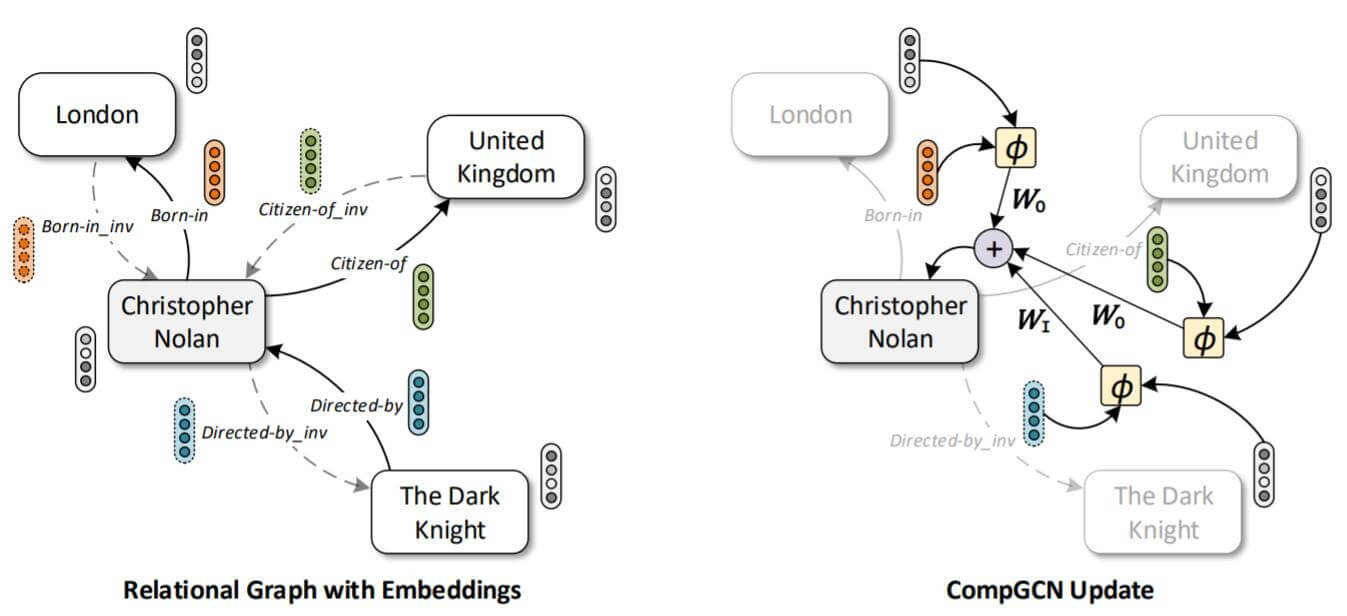### GCN and R - GCN

$$\boldsymbol{H}^{k+1}=f\left(\hat{\boldsymbol{A}} \boldsymbol{H}^{k} \boldsymbol{W}^{k}\right)$$

R - GCN中, 引入了对不同关系$\mathcal{R}$ 的特化处理, 图结构变为了$\mathcal{G}=(\mathcal{V}, \mathcal{R}, \mathcal{E}, \mathcal{X})$:
$$\boldsymbol{H}^{k+1}=f\left(\hat{\boldsymbol{A}} \boldsymbol{H}^{k} \boldsymbol{W}_{r}^{k}\right)$$

$$\left.\mathcal{E}^{\prime}=\mathcal{E} \cup\left\{\left(v, u, r^{-1}\right) \mid(u, v, r) \in \mathcal{E}\right\} \cup\{(u, u, \top) \mid u \in \mathcal{V})\right\}$$

### Relation - Based Composition

$$\boldsymbol{e}_{o}=\phi\left(\boldsymbol{e}_{s}, \boldsymbol{e}_{r}\right)$$

• Subtraction(Sub): $\phi\left(\mathbf{e}_{s}, \mathbf{e}_{r}\right)=\mathbf{e}_{s}-\mathbf{e}_{r}$.
• Multiplication(Mult): $\phi\left(\boldsymbol{e}_{s}, \boldsymbol{e}_{r}\right)=\boldsymbol{e}_{s} \ast \boldsymbol{e}_{r}$.
• Circular - correlation(Corr): $\phi\left(\boldsymbol{e}_{s}, \boldsymbol{e}_{r}\right)=\boldsymbol{e}_{s} \star \boldsymbol{e}_{r}$.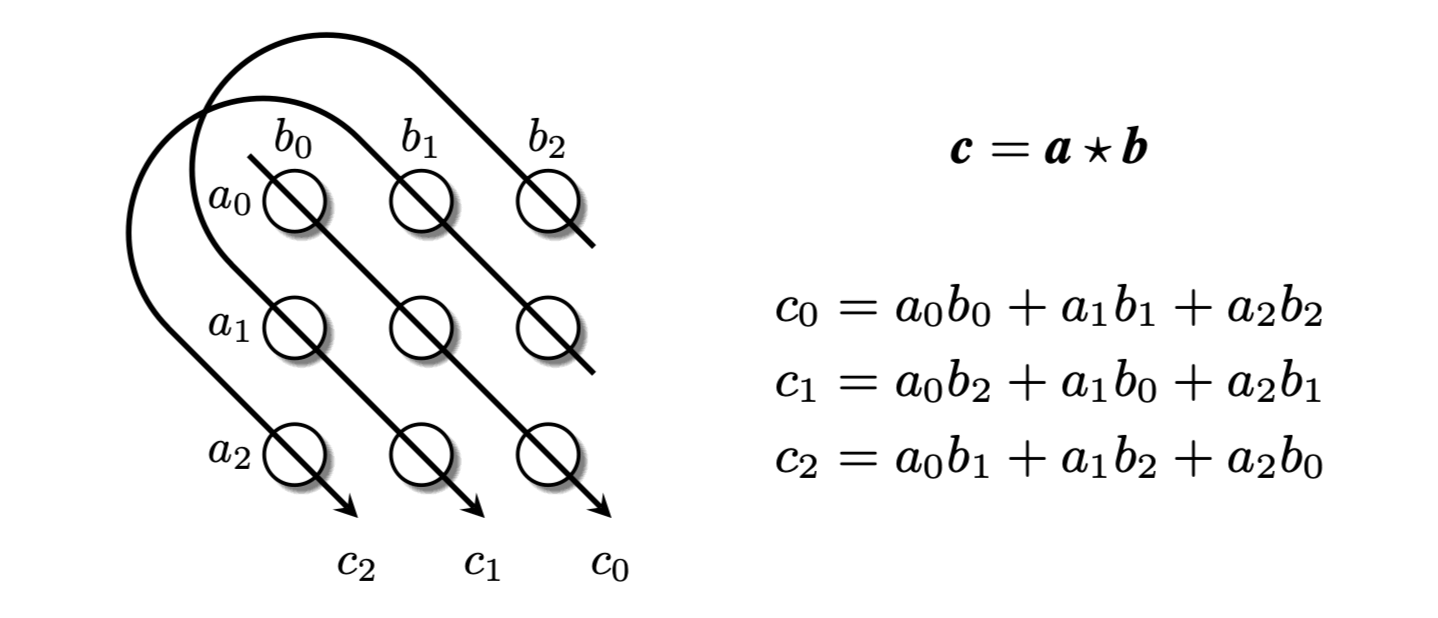### CompGCN Update Equation

$$\boldsymbol{h}_{v}=f\left(\sum_{(u, r) \in \mathcal{N}(v)} \boldsymbol{W}_{r} \boldsymbol{h}_{u}\right)$$

$$\boldsymbol{h}_{v}=f\left(\sum_{(u, r) \in \mathcal{N}(v)} \boldsymbol{W}_{\lambda(r)} \phi\left(\boldsymbol{x}_{u}, \boldsymbol{z}_{r}\right)\right)$$

$$\boldsymbol{W}_{\mathrm{dir}(r)}=\left\{\begin{array}{ll} \boldsymbol{W}_{O}, & r \in \mathcal{R} \\ \boldsymbol{W}_{I}, & r \in \mathcal{R}_{i n v} \\ \boldsymbol{W}_{S}, & r=\top(\text { self-loop }) \end{array}\right.$$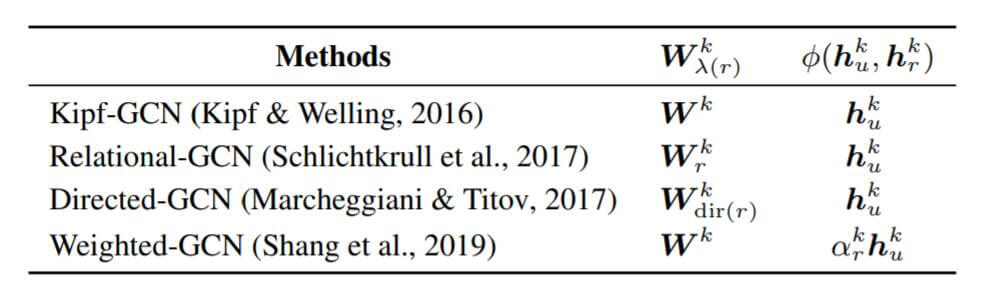$$\boldsymbol{h}_{r}=\boldsymbol{W}_{\mathrm{rel}} \boldsymbol{z}_{r}$$

$\boldsymbol{W_{\text{rel}}}$ 是与关系无关的变换矩阵, 它只是将初始关系嵌入$\boldsymbol{z}_{r}$ 投影到关系嵌入空间而已.

$$\boldsymbol{z}_{r}=\sum_{b=1}^{\mathcal{B}} \alpha_{b r} \boldsymbol{v}_{b}$$

### CompGCN in Multilayer

$$\boldsymbol{h}_{v}^{k+1}=f\left(\sum_{(u, r) \in \mathcal{N}(v)} \boldsymbol{W}_{\lambda(r)}^{k} \phi\left(\boldsymbol{h}_{u}^{k}, \boldsymbol{h}_{r}^{k}\right)\right)$$

$$\boldsymbol{h}_{r}^{k+1}=\boldsymbol{W}_{\mathrm{rel}}^{k} \boldsymbol{h}_{r}^{k}$$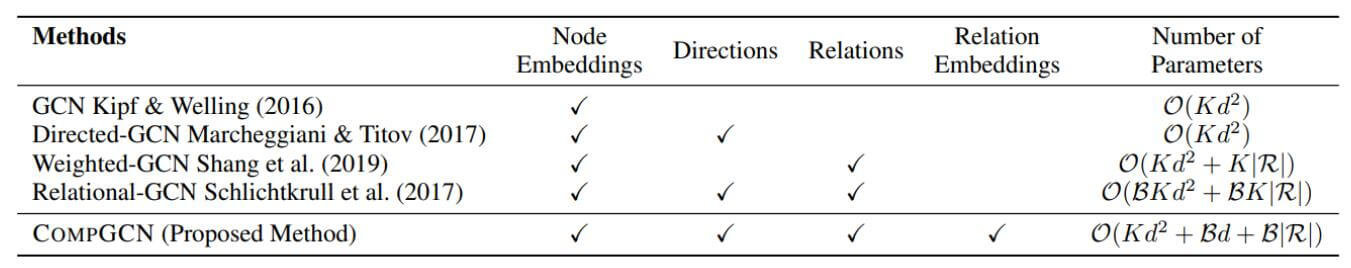$K$ 为GCN的层数, $d$ 为Embedding维度, $\mathcal{B}$ 为基的数量, $\lvert \mathcal{R} \rvert$ 为关系个数.

CompGCN复杂度中的第一项来自于与层数相关的$\boldsymbol{W}_{\text{rel}}, \boldsymbol{W}_{\lambda(r)}$, 第二, 三项分别来自于基函数分解中的基$\boldsymbol{v}_{b}$ 和标量$\alpha_{b r}$.

## Experiments

CompGCN在链接预测上的玩法仍然沿用了R - GCN中Encoder - Decoder的架构

R - GCN使用的Decoder是DistMult, 解码时关系嵌入需要额外训练, 并且存在实体嵌入和关系嵌入的方法不匹配问题, 这就割裂了实体嵌入和关系嵌入的关系. 但CompGCN在编码阶段就引入了关系嵌入, 不会存在关系和实体嵌入训练不匹配的问题: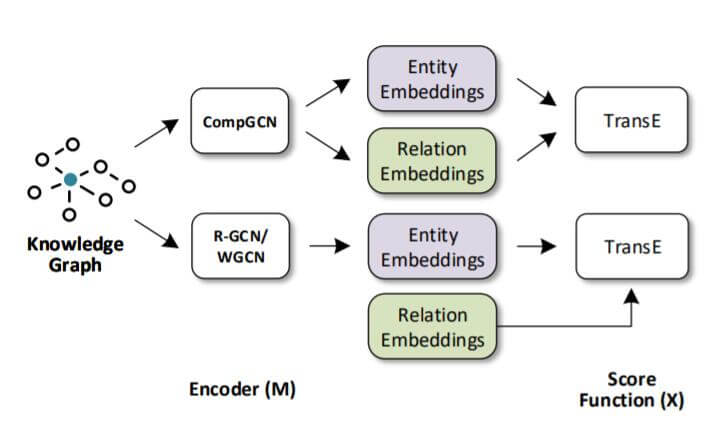#### Main Result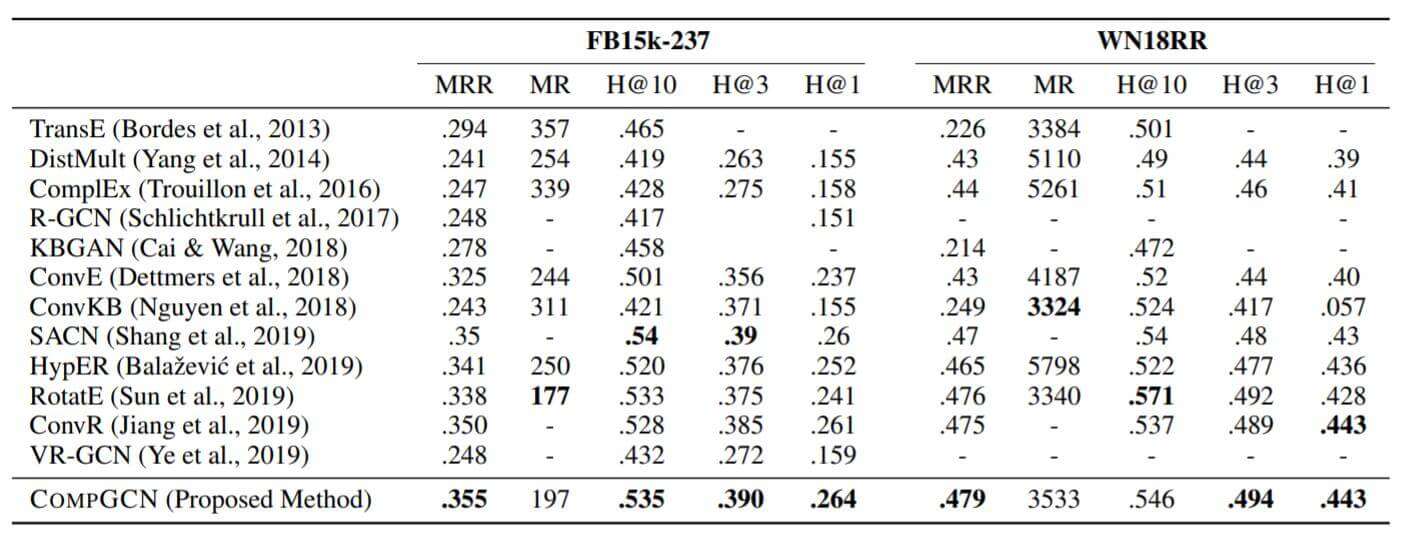#### Comparison in CompGCN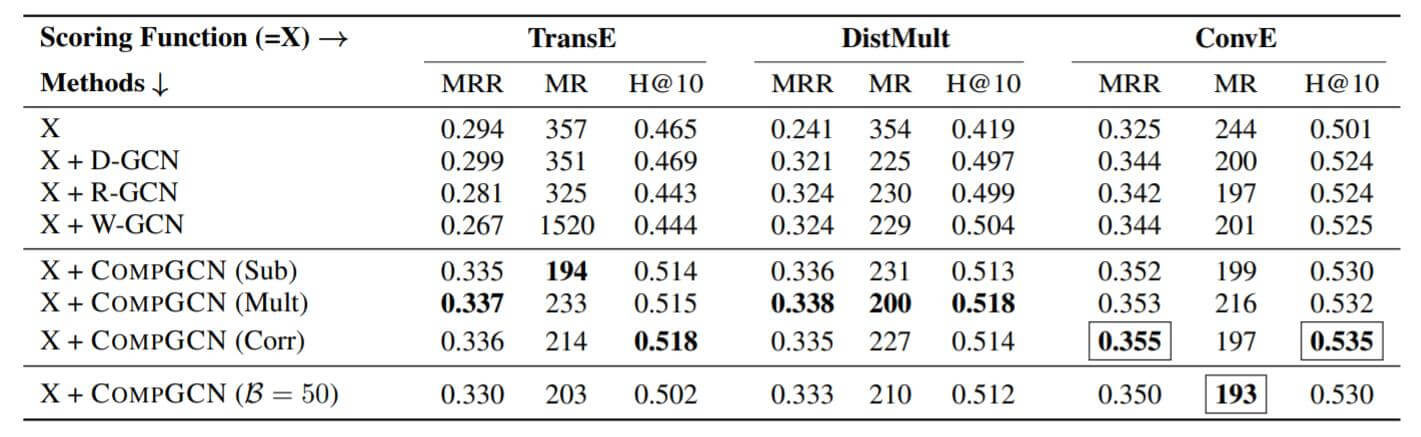#### Scalability of CompGCN

##### Effect of Varying Relation Basis Vectors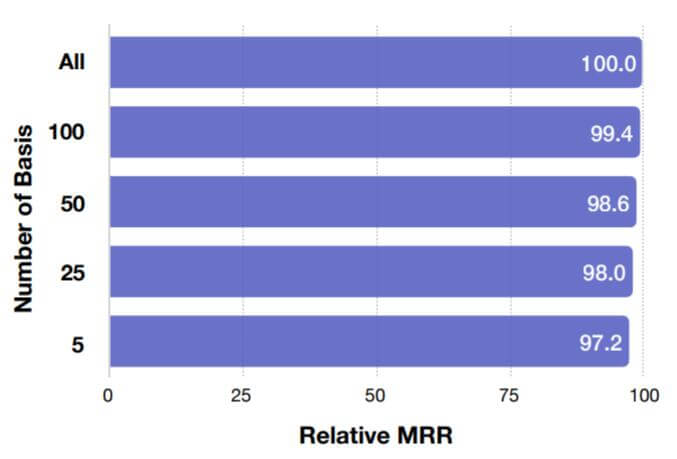##### Effect of Number of Relations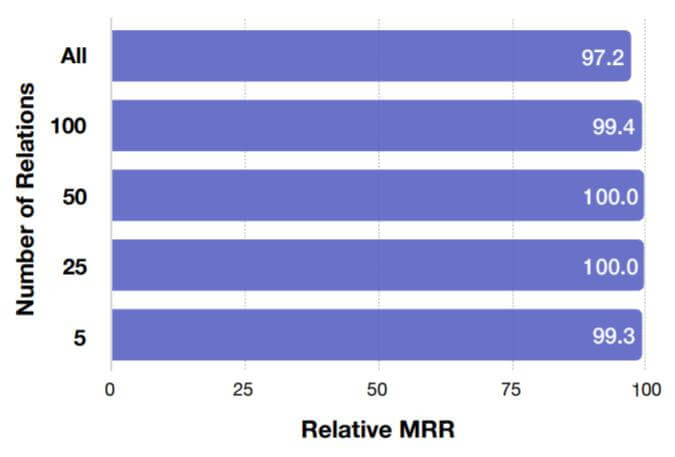##### Comparison with R - GCN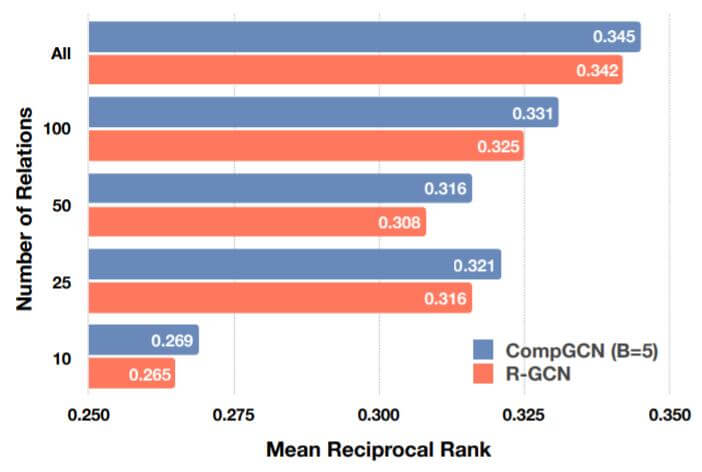### Node and Graph Classification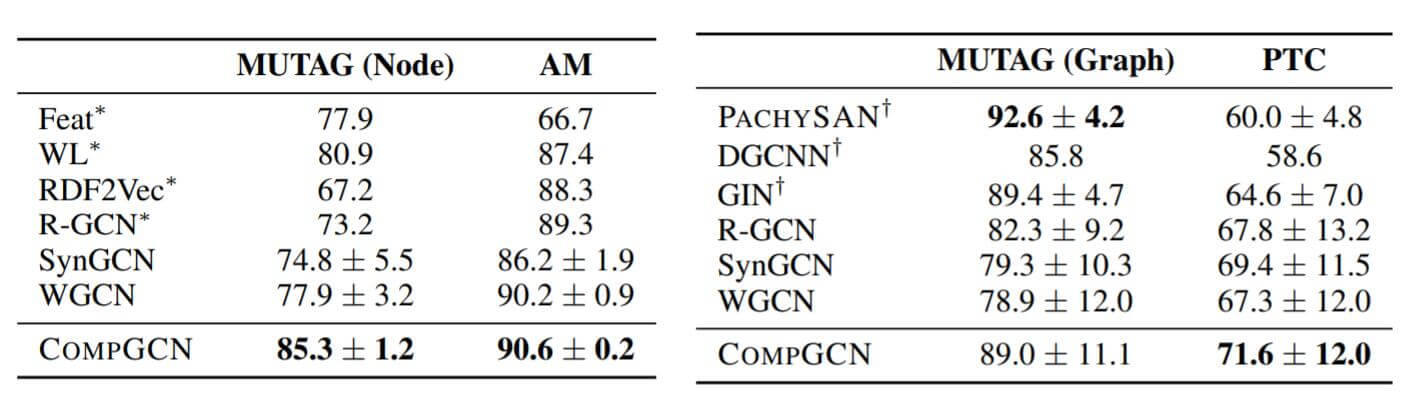## Summary

CompGCN进一步地将Knowledge Embedding中对待关系的方法引入了GCN领域, 在之前的GCN类方法中, 常不将关系嵌入引入, 而在KGE领域中, 将关系和实体嵌入共同使用是非常普遍的.

CompGCN算是集大成者, 作者考虑了很多关于GCN过参数化的内容. 同时兼顾有向, 多关系, 支持关系嵌入三种特性.

上一篇KBAT: Learning Attention-based Embeddings for Relation Prediction in KGs

2021-04-04KEQA: Knowledge Graph Embedding Based Question Answering
Knowledge Graph Embedding Based Question Answering本文是论文Knowledge Graph Embedding Based Question Answering的阅读笔记和个人理解. Bas
2021-03-30
目录